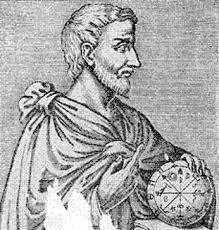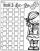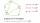# Theorem prove

We want to prove the sentence:

If the natural number n is divisible by six, then n is divisible by three.

From what assumption we started?

x =  6

### Step-by-step explanation:Did you find an error or inaccuracy? Feel free to write us. Thank you!

Showing 1 comment:Math student
How many 15/16 are in 15/16 ?## Related math problems and questions:

• Three numbersHow much we increases the sum of three numbers when the first enlarge by 14, second by 15 and third by 16? Choose any three two-digit numbers and prove results.
• Speed of Slovakian trainsRudolf decided to take the train from the station 'Ostratice' to 'Horné Ozorovce'. In the train timetables found train Os 5409 : km 0 Chynorany 15:17 5 Ostratice 15:23 15:23 8 Rybany 15:27 15:27 10 Dolné Naštice 15:31 15:31 14 Bánovce nad Bebravou 15:35 1
• Proof PTCan you easily prove Pythagoras theorem using Euclidean theorems? If so, do it.
• DivisibilityDetermine the smallest integer which divided 11 gives remainder 4 when divided 15 gives remainder 10 and when divided by 19 gives remainder 16.
• MO C–I–1 2018An unknown number is divisible by just four numbers from the set {6, 15, 20, 21, 70}. Determine which ones.
• Five-digit numberAnna thinks of a five-digit number that is not divisible by three or four. If he increments each digit by one, it gets a five-digit number that is divisible by three. If he reduces each digit by one, he gets a five-digit number divisible by four. If it sw
• Odd/even numberPick any number. If that number is even, divide it by 2. If it's odd, multiply it by 3 and add 1. Now repeat the process with your new number. If you keep going, you'll eventually end up at 1. Every time. Prove. ..
• Air draftThe numbers 1,2,3,4,5 are written on five tickets on the table. Air draft randomly shuffled the tickets and composed a 5-digit number from them. What is the probability that he passed: and, the largest possible number b, the smallest possible number c, a
• Sum of inner anglesProve that the sum of all inner angles of any convex n-angle equals (n-2) . 180 degrees.
• ProveProve that k1 and k2 are the equations of two circles. Find the equation of the line that passes through the centers of these circles. k1: x2+y2+2x+4y+1=0 k2: x2+y2-8x+6y+9=0
• Three digits numberFrom the numbers 1, 2, 3, 4, 5 create three-digit numbers that digits not repeat and number is divisible by 2. How many numbers are there?
• Divisible by nineHow many three-digit natural numbers in total are divisible without a remainder by the number 9?
• Divisible by fiveHow many different three-digit numbers divisible by five can we create from the digits 2, 4, 5? The digits can be repeated in the created number.
• SixteenSixteen minus the quotient of 12 and what number is thirteen?
• DivisibilityIs the number 761082 exactly divisible by 9? (the result is the integer and/or remainder is zero)
• Unknown number 16My number's tens is three times more then ones My number's ones is twice the number of thousands and my number's hundreds is half the number of of tens. I have two ones. which number am I?
• Three-digit integersHow many three-digit natural numbers exist that do not contain zero and are divisible by five?